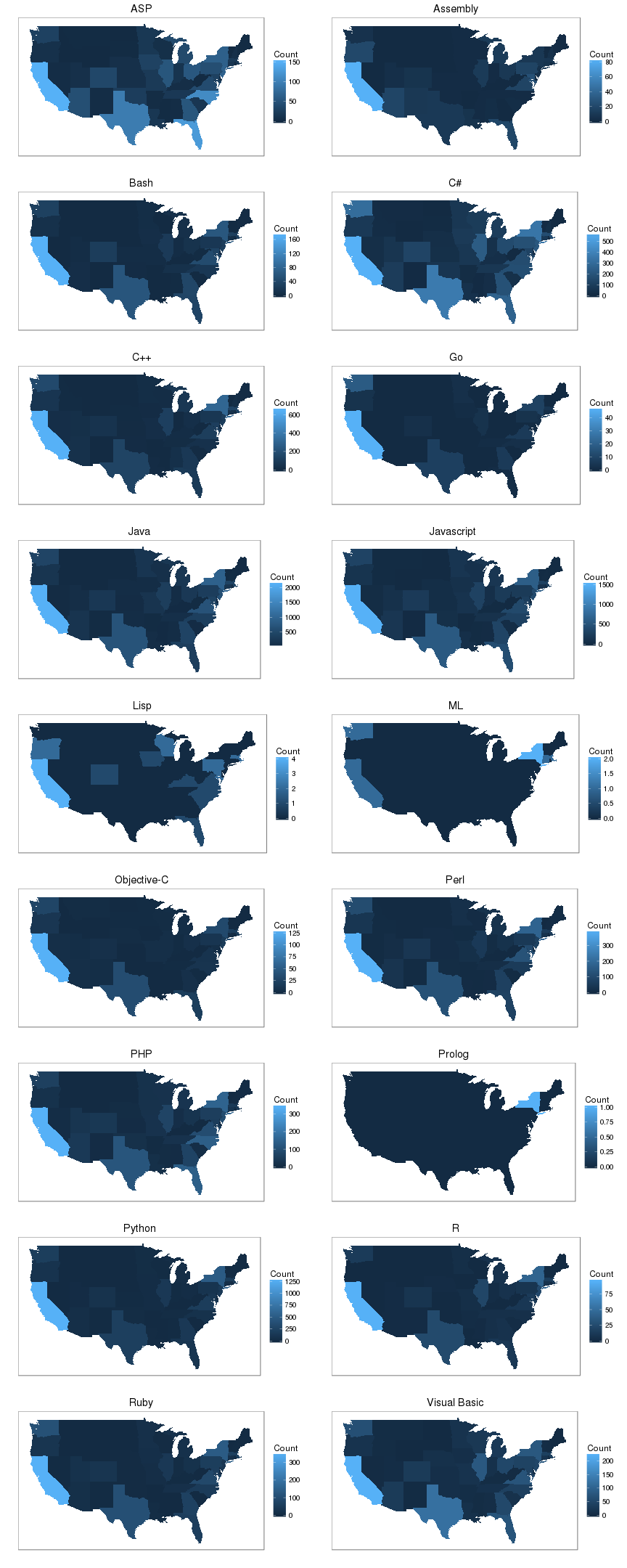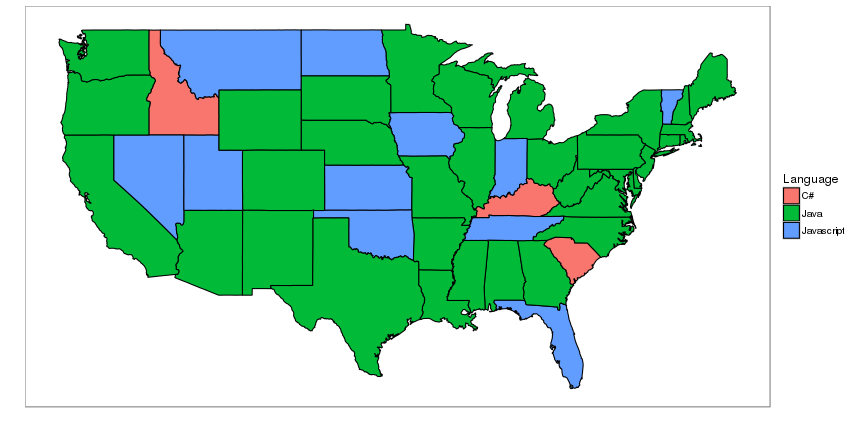# Libraries

Rdicejobs available at https://github.com/BCable/Rdicejobs and installed via devtools:

``````library(Rdicejobs)
``````

Standard libraries available in CRAN:

``````library(ggplot2)
library(grid)
library(maps)
``````

# Create Dataset

``````states <- read.csv("states.csv")
languages <- c(
"C++", "Python", "Ruby", "R", "Objective-C",
"Java", "C#", "Go", "Javascript",
"Lisp", "Perl", "Bash", "Visual Basic",
"ML", "Prolog", "Assembly", "PHP", "ASP"
)
``````

Data gathered on 2016-05-27.

``````states\$Name <- as.character(states\$Name)
states\$Code <- as.character(states\$Code)

first <- TRUE
# loop languages and states
for(lang in languages){
for(i in seq(1, nrow(states))){
# get data for one state and one language
lang_count <- dice_job_count(
skill=lang, country="US", state=states[i,"Code"]
)

# create vectors
write.table(
data.frame(
State.Name=states[i,"Name"],
State.Code=states[i,"Code"],
Language=lang,
Count=lang_count
), "dice.csv", sep=",",
row.names=FALSE, col.names=first, append=!first
)

first <- FALSE
}
}
``````

# Load and Gather Data

``````df <- read.csv("dice.csv")
``````
``````states_map <- map_data("state")
``````
``````names(df) <- c("state", "code", "language", "Count")
df\$state <- tolower(df\$state)
``````
``````maxes <- as.vector(tapply(df\$Count, df\$state, max))
df_grouped <- df[order(df\$state),]
df_grouped\$Max <- rep(maxes, each=length(levels(df\$language)))
df_grouped <- df_grouped[df_grouped\$Count != 0,]
df_grouped <- df_grouped[
df_grouped\$Count == df_grouped\$Max,c("state","language")
]
names(df_grouped) <- c("state", "Language")
all_states <- levels(as.factor(df\$state))
no_data_states <- all_states[!all_states %in% df_grouped\$state]
df_grouped <- rbind(df_grouped, data.frame(
state=no_data_states,
Language=rep("No Data", length(no_data_states))
))
``````

# Plotting Functions

``````theme_plot <- function(g){
g <- g + theme_bw() + theme(
panel.grid=element_blank(),
axis.text=element_blank(), axis.ticks=element_blank()
) + xlab("") + ylab("")
g
}
``````
``````map_plotter <- function(language, plot_row, plot_col){
df_filter <- df[df\$language == language,]

g <- ggplot(df_filter, aes(map_id=state))
g <- g + geom_map(aes(fill=Count), map=states_map)
g <- g + expand_limits(x=states_map\$long, y=states_map\$lat)
g <- g + ggtitle(language)
plot(theme_plot(g), vp=viewport(
layout.pos.row=plot_row,
layout.pos.col=plot_col
))
}
``````

# Plot All Languages

``````total_lang <- length(levels(df\$language))
total_cols <- 2
total_rows <- total_lang/total_cols
grid.newpage()
pushViewport(viewport(layout=grid.layout(total_rows, total_cols)))
for(i in 1:total_lang){
cur_row <- ceiling(i/total_cols)
cur_col <- (i+1)%%2+1
map_plotter(sort(levels(df\$language))[i], cur_row, cur_col)
}
``````# Plot Overall Data

``````g <- ggplot(df_grouped, aes(map_id=state))
g <- g + geom_map(aes(fill=Language), color="black", map=states_map)
g <- g + expand_limits(x=states_map\$long, y=states_map\$lat)
theme_plot(g)
``````# Results

Looking at the frequency graphs, a strong trend in programming jobs in California can be seen pretty clearly. Texas is also a strong consistent second place, and there seems to be a steady distribution of a decent amount of jobs all up the east coast. The northwest parts (east of Oregon/Washington) show very little job activity, which is to be expected since those states such as Idaho, Montana, and South/North Dakota have very lower populations in general due to the geography of the country.

That being said, this data seems to be highly biased towards California. This could be indicative of general IT jobs in the state due to Silicon Valley, but it could also be an indication that Dice itself is just one data source and perhaps they market heavier towards Silicon Valley.

The overall map shows that the prevailing programming job market is in Java.